# Non-commutative anomaly

(diff) ← Older revision | Latest revision (diff) | Newer revision → (diff)

Many fundamental calculations of quantum field theory reduce, in essence, to the computation of the determinant of some operator. One could even venture to say that, at one-loop order, any such theory reduces to a theory of determinants. It has been realized recently (2000) that the calculation of effective actions through the corresponding determinants is not free from dangers (which were overlooked till very recently by the physics community) stemming from the existence of a multiplicative anomaly (also non-commutative anomaly or determinant anomaly), i.e., the fact that the determinant of a product of operators is not equal, in general, to the product of the determinants. The difference proves to be physically relevant, since only in some particular cases can it be simply absorbed into the renormalized constants. The operators involved are "differential" ones, as the normal physicist would say. In fact, properly speaking, they are pseudo-differential operators (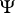DOs; cf. also Pseudo-differential operator), that is, in loose terms "some analytic functions of differential operators" (such as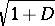or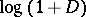, but not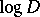). This is explained in detail in [a3].

Important as the concept of determinant of a differential or pseudo-differential operator may be for theoretical physicists, it is surprising that this seems not to be a subject of study among function analysts or mathematicians in general. The question of regularizing infinite determinants was already addressed by K. Weierstrass in a way that, although it has been pursued by some theoretical physicists with success, is not without problems (as a general method) since it ordinarily leads to non-local contributions that cannot be given a physical meaning in quantum field theory. One should mention, for completion, that there are, since long ago, well-established theories of determinants for degenerate operators, for trace-class operators in a Hilbert space, for Fredholm operators, etc. but, again, these definitions of determinant do not fulfil all the needs mentioned above which arise in quantum field theory.

Take the determinant of the Dirac operator. It is defined roughly as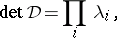where the infinite product is regularized with (for example) zeta-function or Pauli–Villars regularization (cf. also Zeta-function method for regularization). The zeta-function definition of the determinantis possibly the one that has more firm mathematical grounds [a1], [a2]. In spite of starting from the identity, it is known to develop a multiplicative anomaly: the determinant of the product of two operators is not equal, in general, to the product of the determinants (even if the operators commute!). This happens already with very simple operators (as two one-dimensional harmonic oscillators only differing in a constant term, Laplacians plus different mass terms, etc.) [a4].

Given pseudo-differential operators,and, even if their zeta-functions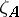,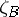and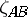exist it turns out that, in general,. The non-commutative anomaly is defined as: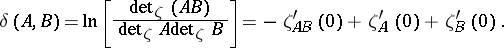The Wodzicki formula for the multiplicative anomaly is very useful. It reads [a4]: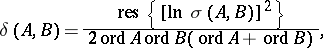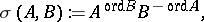in terms of the Wodzicki residue.

How to Cite This Entry:
Non-commutative anomaly. Encyclopedia of Mathematics. URL: http://encyclopediaofmath.org/index.php?title=Non-commutative_anomaly&oldid=14315
This article was adapted from an original article by E. Elizalde (originator), which appeared in Encyclopedia of Mathematics - ISBN 1402006098. See original article